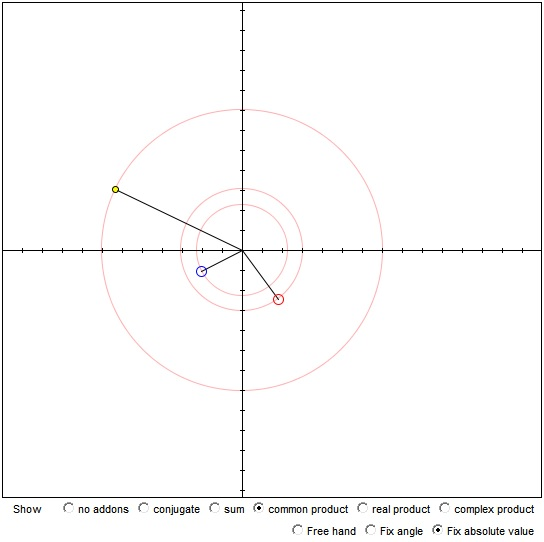# Complex Numbers A Dynamic Tool

This is just a short refresher of what is a complex number and a tool that helps investigate some of their properties and the operations

Complex number is an ordered pair (x, y) of real numbers. It can also be written in the algebraic form as

(x, y) = x + yi.

(x1, y1) + (x2, y2) = (x1 + x2, y1 + y2),

but are multiplied in a more intricate way:

(x1, y1)(x2, y2) = (x1x2 - y1y2, x1y2 + x2y1).

This is the common operation of multiplication. There are two more that can be defined. One is the real product, the product of two complex numbers that is always real, the other is complex product, the product of complex numbers that is alway complex. For two complex numbers zk, k = 1, 2, the real product is defined as

z1·z2 = (z1·Conj(z2) + z1)·z2)/2

and the complex product as

z1×z2 = (z1·Conj(z2) - Conj(z1)·z2)/2.

### If you are reading this, your browser is not set to run Java applets. Try IE11 or Safari and declare the site https://www.cut-the-knot.org as trusted in the Java setup.What if applet does not run?

### Worthy Observations

1. The conjugate Conj(z) is a reflection of z in the real axis. This explains why Conj(Conj(z) = z.

2. Like vectors, complex numbers are added according the parallelogram rule.

3. When one of two complex numbers is fixed and the other traces a straight line their sum also traces a straight line. The two lines are parallel.

4. When two complex numbers have fixed modules, so does their (common) product.

5. Multiplying a complex number by a real number (i.e. dragging one of the dots along the x-axis) does not change the argument of the number but only its module.

6. Multiplication by a purely complex number (i.e., the one lying on the y-axis) rotates a number by 90o counterclockwise.

7. Multiplication by -1 reflects a number in the origin.

8. The real product of two numbers is always real: it always lies on the x-axis. It is zero when the radius-vectors of the two numbers are perpendicular.

9. The complex product of two numbers is always complex: it always lies on the y-axis. It is zero when the two numbers are collinear with the origin, i.e., when their quotient is real.### Complex Numbers